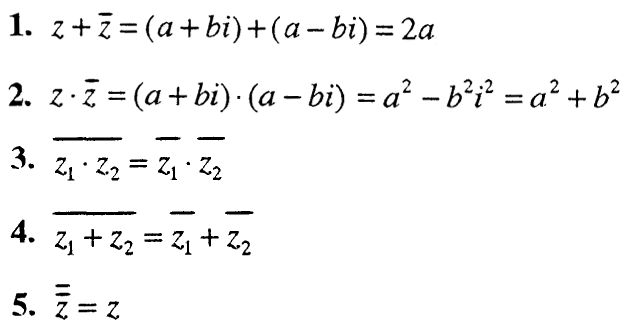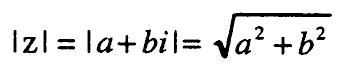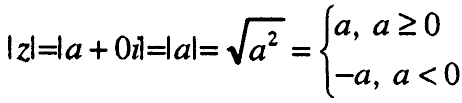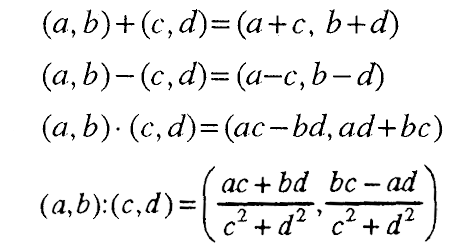# Complex numbers

The symbol i is a number whose square is -1, ie. i² = -1

The number i is called an imaginary unit. Numbers of the form a + bi, where a, b ∊ R, and i-imaginary unit, are called complex numbers.

The set of complex numbers is denoted by C = {a + bi | a, b ∈ R}.

The notation a + bi is called the algebraic or standard form of a complex number.
The number a is called the real part, and b – the imaginary part of the complex number, ie.
a = Re (Z), b = Im (Z), z = a + bi.
For the complex number z = a + bi we adopt:
– if b = 0, then a + bi = a is a real number;
– if b ≠ 0, then a + bi is called an imaginary (unreal) number;
– if b ≠ 0 and a = 0, then a + bi is called a purely imaginary number.

For the complex numbers a + bi and c + di we say that they are equal if their real parts are equal and their imaginary parts are equal, ie.
a + bi = c + di <=>
a = c ⋀ b = d

## 1. COMPLEX NUMBER OPERATIONS

#### 1.1. ZBIR

(a + bi) + (c + di) = (a + c) + (b + d) i

Example: (2 + 4i) + (2 – 3i) = 2 + 4i + 2 – 3i = 4 + i

___________________________________________

#### 1.2. PRODUCT

(a + bi) · (c + di) = (ac – bd) + (ad + bc) i

Example:
(2 – 3i) · (5 + 2i) = 10 – 15i + 4i – 6i² =
= 10 – 15i + 4i -6 · (-1) = 10 – 15i + 4i +6 = 16 -11i

__________________________________________

#### 1.3. DIFFERENCE

(a + bi) – (c + di) = (a – c) + (b – d) i
The complex number -z = -a – bi is the opposite of the number z = a + bi, because (a + bi) + (-a – bi) = 0.

Example:
(2 + 4i) – (6 – i) = 2 + 4i – 6 + i = -4 + 5j

_______________________________________

#### 1.4. QUICK

The complex number z = a – bi is a conjugate complex number of the number z = a + bi. The following properties apply to conjugate complex numbers z and :z:For the quotient of the complex numbers a + bi and c + di we use the rule:The quotient of two complex numbers can be obtained through the following procedure:_______________________________________

#### 1.5. ACCELERATION OF COMPLEX NUMBER

The definition of a complex number z is defined as the gradation of a real number, ie.

z¹ = z
zⁿ⁺¹ = zⁿ ∙ z
z⁰ = 1
z⁻ⁿ = (z⁻¹) ⁿ

For the degrees of the imaginary unit i applies:
iⁿ ∈ {1, -1, i, -i}, means

i¹ = i
i² = -1
i³ = -i
i⁴ = 1

Example:
i³⁹⁷ = i⁴͘͘͘͘͘ ⁹⁷⁺³ = i³ = -i

## 2. Complex number module

The modulus or absolute value of a complex number z = a + bi is called the real negative number and is denoted by | Z |If the complex number a + bi is a real number, ie. if b = 0, then:Since a² + b² = (a + bi) · (a – bi), the equation is true:
͞Z ∙ z = | z | ²

The following applies to the complex numbers z₁ and z₂:3. COMPLEX NUMBERS AS SUBJECTED DOUBLE NUMBERS

The ordered pair of real numbers z = (a, b) = a + bi is a complex number, ie.

C = {(a, b) | a, b ∊ R}

The operations of addition, subtraction, multiplication and division are performed according to the following rules:For complex numbers (a, b) and (c, d) the following applies:

(a, b) = (c, d) <=> a = c b = d

A complex number (0, 0) is called a complex zero.

For the complex number of imaginary units, ie. i = (0, 1). 3that any complex number z = a + bi can be written as follows:

z = a + bi = (a, b) = (a, 0) + (b, 0) · (0, 1)

Total Page Visits: 1187 - Today Page Visits: 1